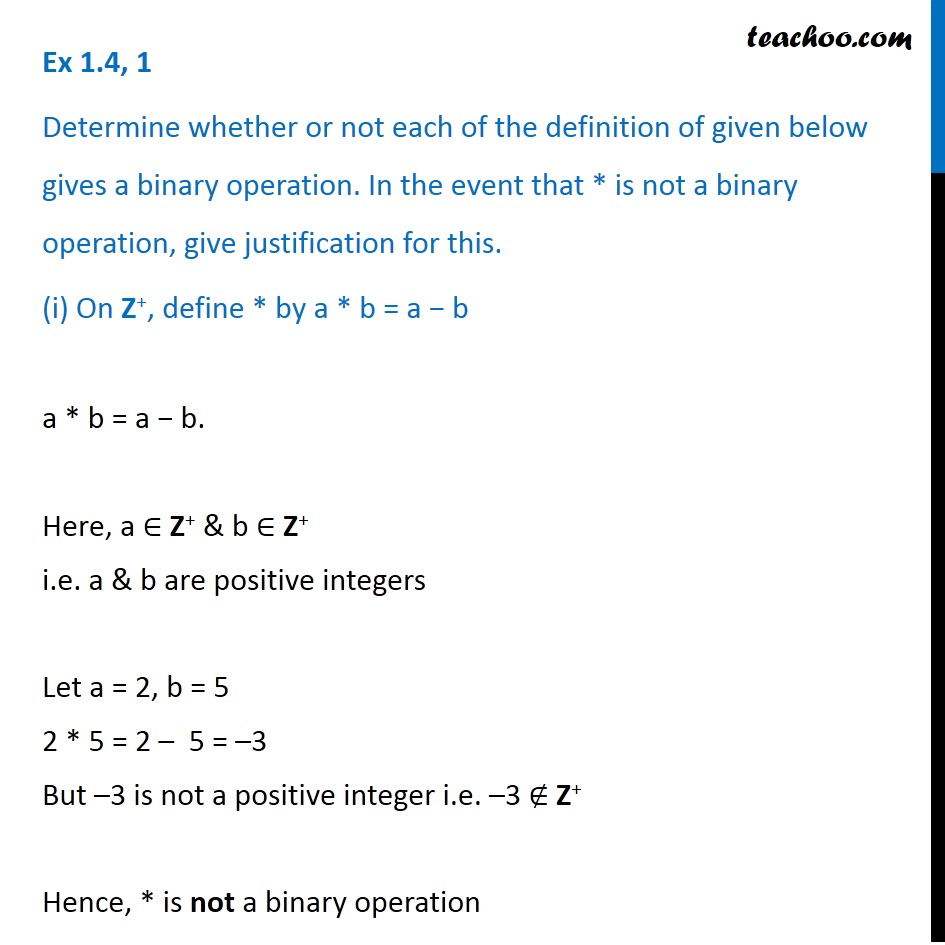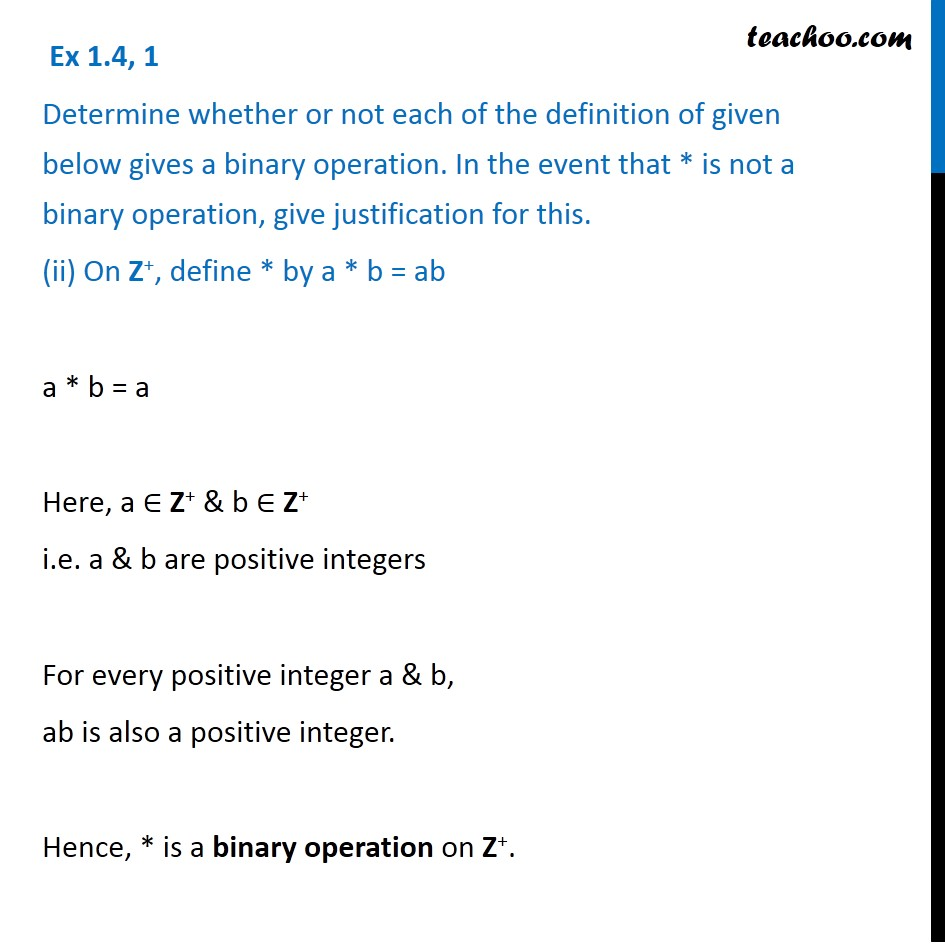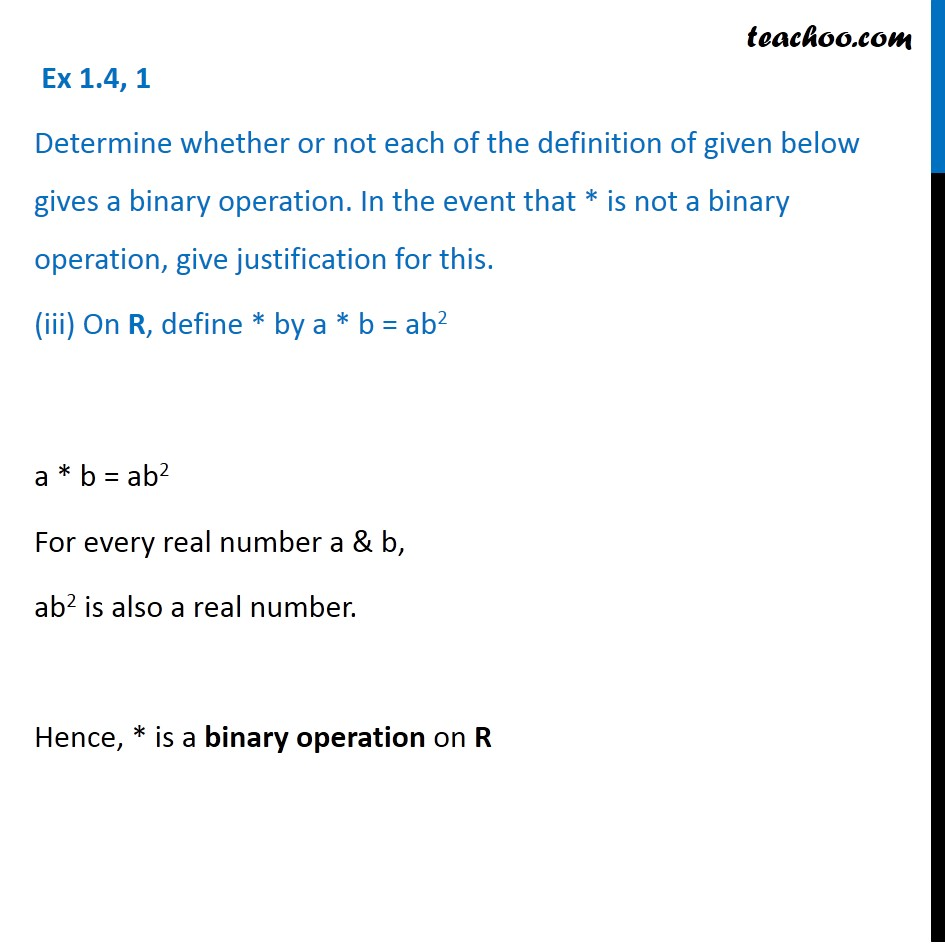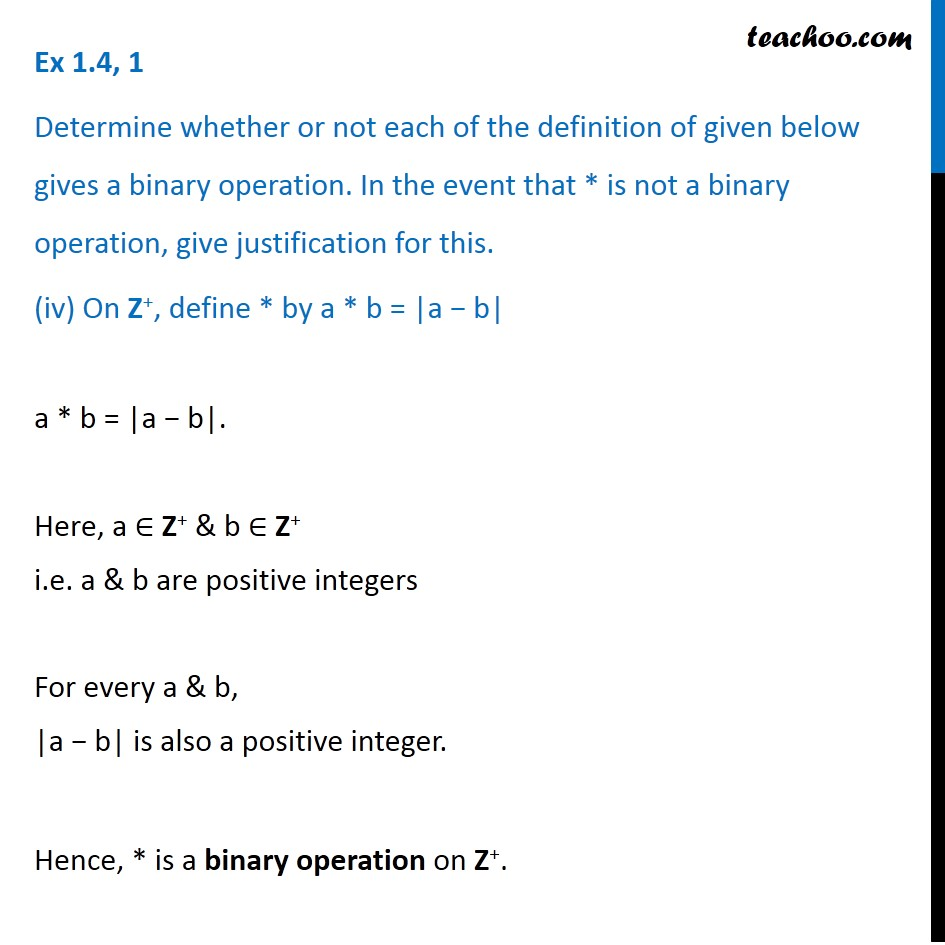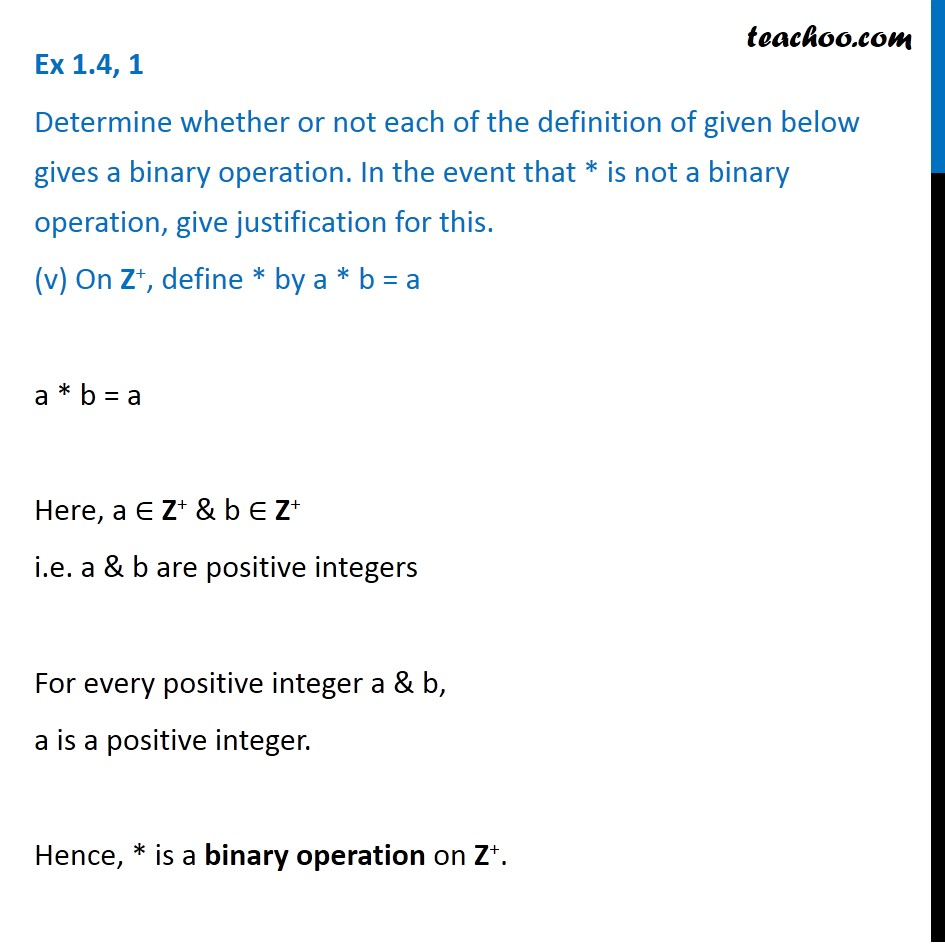1. Chapter 1 Class 12 Relation and Functions
2. Serial order wise
3. Ex 1.4

Transcript

Ex 1.4, 1 Determine whether or not each of the definition of given below gives a binary operation. In the event that * is not a binary operation, give justification for this. (i) On Z+, define * by a * b = a − b a * b = a − b. Here, a ∈ Z+ & b ∈ Z+ i.e. a & b are positive integers Let a = 2, b = 5 2 * 5 = 2 – 5 = –3 But –3 is not a positive integer i.e. –3 ∉ Z+ Hence, * is not a binary operation Ex 1.4, 1 Determine whether or not each of the definition of given below gives a binary operation. In the event that * is not a binary operation, give justification for this. (ii) On Z+, define * by a * b = ab a * b = a Here, a ∈ Z+ & b ∈ Z+ i.e. a & b are positive integers For every positive integer a & b, ab is also a positive integer. Hence, * is a binary operation on Z+. Ex 1.4, 1 Determine whether or not each of the definition of given below gives a binary operation. In the event that * is not a binary operation, give justification for this. (iii) On R, define * by a * b = ab2 a * b = ab2 For every real number a & b, ab2 is also a real number. Hence, * is a binary operation on R Ex 1.4, 1 Determine whether or not each of the definition of given below gives a binary operation. In the event that * is not a binary operation, give justification for this. (iv) On Z+, define * by a * b = |a − b| a * b = |a − b|. Here, a ∈ Z+ & b ∈ Z+ i.e. a & b are positive integers For every a & b, |a − b| is also a positive integer. Hence, * is a binary operation on Z+. Ex 1.4, 1 Determine whether or not each of the definition of given below gives a binary operation. In the event that * is not a binary operation, give justification for this. (v) On Z+, define * by a * b = a a * b = a Here, a ∈ Z+ & b ∈ Z+ i.e. a & b are positive integers For every positive integer a & b, a is a positive integer. Hence, * is a binary operation on Z+.

Ex 1.4

About the AuthorDavneet Singh
Davneet Singh is a graduate from Indian Institute of Technology, Kanpur. He has been teaching from the past 10 years. He provides courses for Maths and Science at Teachoo.